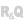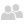HelpEditorsUML editorObject diagramOverviewElements of an object diagramSee alsoActivity diagramClass diagramCollaboration diagramComponent diagramDeployment diagramGenerating a class diagramGeneration of a class diagram using reverse engineeringObject diagramOperations performed on activity diagramsOperations performed on class diagramsOperations performed on collaboration diagramsOperations performed on component diagramsOperations performed on deployment diagramsOperations performed on object diagramsOperations performed on sequence diagramsOperations performed on state-transition diagramsOperations performed on use case diagramsSequence diagramState-transition diagramThe UML modelUse case diagram
 OverviewElements of an object diagram WINDEVWEBDEVWINDEV MobileOthersObject diagram Overview An object diagram represents a set of objects and how they relate to each other at a certain moment in time.An object diagram is used to show a context (before or after an interaction between objects, for example).For example, the diagram below presents a section of the general structure of motorcycles:Elements of an object diagram An object diagram includes the following elements:object: represents a class instance.Remark: If a class diagram is opened, you can create an object from a class in this diagram (Drag and drop it from the "UML analysis" pane).composite object: visually represents an object made of other objects. For example: a window that contains scrollbars, buttons, etc.link: represents the relationship between different objects.UML package: divides and organizes the diagram representation (in the same way that directories organize files). Each package can contain objects and composite objects.For more details on the actions available on object diagrams, see Operations performed on object diagrams. Minimum version required Version 9 This page is also available for…